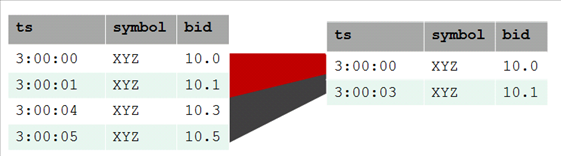# TIMESERIES clause and aggregates

The SELECT..TIMESERIES clause and time series aggregates help solve the problem of gaps in input records by normalizing the data into 3-second time slices and interpolating the bid price when it finds gaps.

The SELECT..TIMESERIES clause and time series aggregates help solve the problem of gaps in input records by normalizing the data into 3-second time slices and interpolating the bid price when it finds gaps.

## TIMESERIES clause

The TIMESERIES clause is an important component of time series analytics computation. It performs gap filling and interpolation (GFI) to generate time slices missing from the input records. The clause applies to the timestamp columns/expressions in the data, and takes the following form:

``````TIMESERIES slice_time AS 'length_and_time_unit_expression'
OVER ( ... [ window-partition-clause[ , ... ] ]
... ORDER BY time_expression )
... [ ORDER BY table_column [ , ... ] ]
``````

## Time series aggregate functions

The Timeseries Aggregate (TSA) functions TS_FIRST_VALUE and TS_LAST_VALUE evaluate the values of a given set of variables over time and group those values into a window for analysis and aggregation.

TSA functions process the data that belongs to each time slice. One output row is produced per time slice or per partition per time slice if a partition expression is present.

The following table shows 3-second time slices where:

• The first two rows fall within the first time slice, which runs from 3:00:00 to 3:00:02. These are the input rows for the TSA function's output for the time slice starting at 3:00:00.

• The second two rows fall within the second time slice, which runs from 3:00:03 to 3:00:05. These are the input rows for the TSA function's output for the time slice starting at 3:00:03.

The result is the start of each time slice.## Examples

The following examples compare the values returned with and without the TS_FIRST_VALUE TSA function.

This example shows the TIMESERIES clause without the TS_FIRST_VALUE TSA function.

``````=> SELECT slice_time, bid FROM TickStore TIMESERIES slice_time AS '3 seconds' OVER(PARTITION by TickStore.bid ORDER BY ts);
``````

This example shows both the TIMESERIES clause and the TS_FIRST_VALUE TSA function. The query returns the values of the `bid` column, as determined by the specified constant interpolation scheme.

``````=> SELECT slice_time, TS_FIRST_VALUE(bid, 'CONST') bid FROM TickStore
TIMESERIES slice_time AS '3 seconds' OVER(PARTITION by symbol ORDER BY ts);
``````

Vertica interpolates the last known value and fills in the missing datapoint, returning 10 at 3:00:03:

 First query Interpolated value `````` slice_time | bid ---------------------+----- 2009-01-01 03:00:00 | 10 2009-01-01 03:00:03 | 10.5 (2 rows) `````` `==>` ``` slice_time | bid ---------------------+----- 2009-01-01 03:00:00 | 10 2009-01-01 03:00:03 | 10 (2 rows) ```